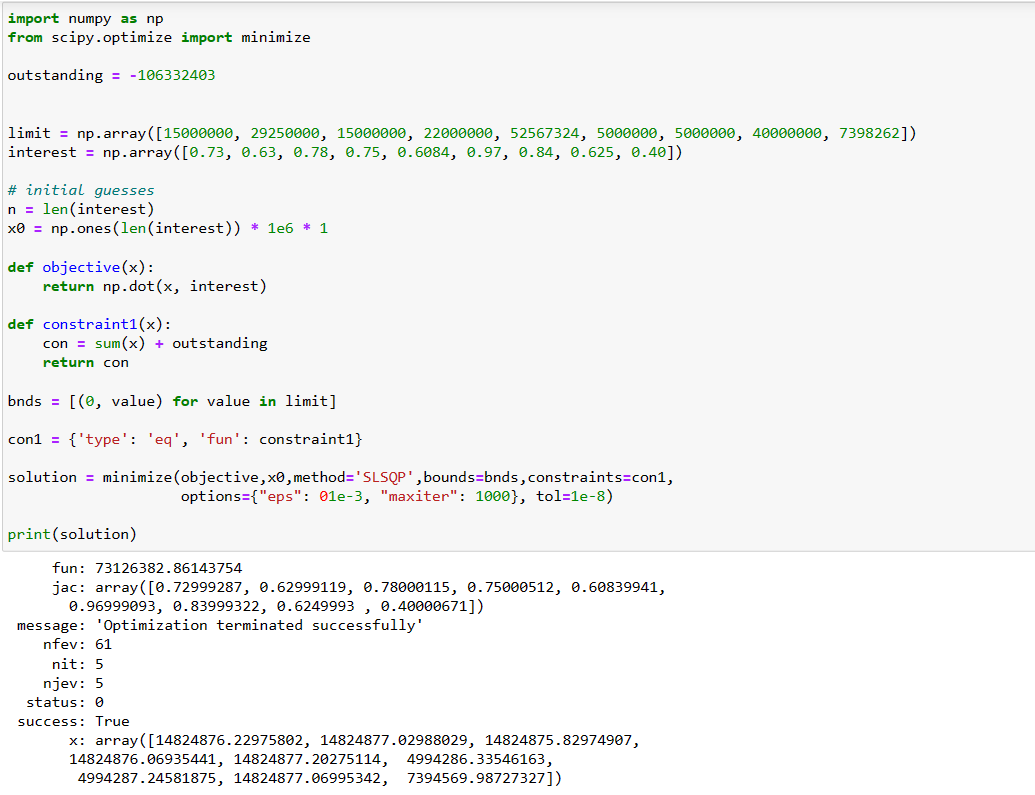# Optimizing a function with constraints using SciPy's minimize function with constraintsby kanikaUpdated: Mar 29, 2023Solution Kit

Optimizing a function with constraints is the process of finding the maximum or minimum value of a function while satisfying certain restrictions or limitations. This is done by using methods such as Lagrange multipliers, which allow one to find the optimal solution while taking into account the constraints that are imposed. The goal of optimizing a function with constraints is to find the best solution to a problem given the restrictions or constraints that are imposed.

minimize function is a function that attempts to minimize a scalar function of one or more variables by using a variety of algorithms. It is a part of the SciPy library, which is a collection of scientific computing tools for Python. The minimize function is used to find the minimum of a given function, often used in optimization problems.

Here is an example of optimizing a function with constraints using SciPy's minimize function with constraints.Fig 1: Preview of the output that you will get on running this code from your IDE

### Code

In this solution, we use the minimizing function of the SciPy library.

### Instructions

Follow the steps carefully to get the output easily.

1. Install Jupyter Notebook on your computer.
2. Open terminal and install the required libraries with following commands.
3. Install numpy - pip install numpy.
4. Install SciPy - pip install scipy.
5. Copy the snippet using the 'copy' button and paste it into that file.
6. Run the file using run button.

I hope you found this useful. I have added the link to dependent libraries, and version information in the following sections.

I found this code snippet by searching for "Optimizing a function with constraints using SciPy's minimize function with constraints" in kandi. You can try any such use case!

### Dependent Libraries﻿

scipyby scipy

Python11340Version:v1.11.0rc1SciPy library main repository

Support
Quality
Security
Reuse

scipyby scipy

Python11340Version:v1.11.0rc1License: Permissive (BSD-3-Clause)

SciPy library main repository
Support
Quality
Security
Reuse

If you do not have SciPy that is required to run this code, you can install it by clicking on the above link and copying the pip Install command from the scipy page in kandi.

You can search for any dependent library on kandi like scipy

### Environment Tested

I tested this solution in the following versions. Be mindful of changes when working with other versions.

1. The solution is created in Python 3.9.6
2. The solution is tested on SciPy version 1.9.3

Using this solution, we are able to solve linear equations using scipy's linalg.solve function. It can also be used for finding the inverse of a matrix or calculating the determinant of a matrix.

### Support

1. For any support on kandi solution kits, please use the chat
2. For further learning resources, visit the Open Weaver Community learning page.

See similar Kits and Libraries